Next: Converting from audio signals Up: Audio and control computations Previous: Control   Contents   Index

# Control streams

Control computations may come from a variety of sources, both internal and external to the overall computation. Examples of internally engendered control computations include sequencing (in which control computations must take place at pre-determined times) or feature detection of the audio output (for instance, watching for zero crossings in a signal). Externally engendered ones may come from input devices such as MIDI controllers, the mouse and keyboard, network packets, and so on. In any case, control computations may occur at irregular intervals, unlike audio samples which correspond to a steadily ticking sample clock.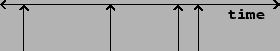We will need a way of describing how information flows between control and audio computations, which we will base on the notion of a control stream. This is simply a collection of numbers--possibly empty--that appear as a result of control computations, whether regularly or irregularly spaced in logical time. The simplest possible control stream has no information other than a time sequence:Although the time values are best given in units of samples, their values aren't quantized; they may be arbitrary real numbers. We do require them to be sorted in nondecreasing order:Each item in the sequence is called an event.

Control streams may be shown graphically as in Figure 3.3. A number line shows time and a sequence of arrows points to the times associated with each event. The control stream shown has no data (it is a time sequence). If we want to show data in the control stream we will write it at the base of each arrow.

A numeric control stream is one that contains one number per time point, so that it appears as a sequence of ordered pairs:where the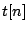are the time points and the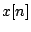are the signal's values at those times.

A numeric control stream is roughly analogous to a MIDI controller", whose values change irregularly, for example when a physical control is moved by a performer. Other control stream sources may have higher possible rates of change and/or more precision. On the other hand, a time sequence might be a sequence of pedal hits, which (MIDI implementation notwithstanding) shouldn't be considered as having values, just times.

Numeric control streams are like audio signals in that both are just time-varying numeric values. But whereas the audio signal comes at a steady rate (and so the time values need not be specified per sample), the control stream comes unpredictably--perhaps evenly, perhaps unevenly, perhaps never.

Let us now look at what happens when we try to convert a numeric control stream to an audio signal. As before we'll choose a block size. We will consider as a control stream a square wave of period 5.5:and demonstrate three ways it could be converted to an audio signal. Figure 3.4 (part a) shows the simplest, fast-as-possible, conversion. Each audio sample of output simply reflects the most recent value of the control signal. So samples 0 through 3 (which are computed at logical time 4 because of the block size) are 1 in value because of the point (2, 1). The next four samples are also one, because of the two points, (4.75, 0) and (7.5, 1), the most recent still has the value 1.

Fast-as-possible conversion is most appropriate for control streams which do not change frequently compared to the block size. Its main advantages are simplicity of computation and the fastest possible response to changes. As the figure shows, when the control stream's updates are too fast (on the order of the block size), the audio signal may not be a good likeness of the sporadic one. (If, as in this case, the control stream comes at regular intervals of time, we can use the sampling theorem to analyze the result. Here the Nyquist frequency associated with the block rateis lower than the input square wave's frequency, and so the output is aliased to a new frequency lower than the Nyquist frequency.)Part (b) shows the result of nearest-sample conversion. Each new value of the control stream at a time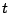affects output samples starting from index(the greatest integer not exceeding). This is equivalent to using fast-as-possible conversion at a block size of 1; in other words, nearest-sample conversion hides the effect of the larger block size. This is better than fast-as-possible conversion in cases where the control stream might change quickly.

Part (c) shows sporadic-to-audio conversion, again at the nearest sample, but now also using two-point interpolation to further increase the time accuracy. Conceptually we can describe this as follows. Suppose the value of the control stream was last equal to, and that the next point is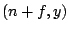, whereis an integer andis the fractional part of the time value (so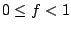). The first point affected in the audio output will be the sample at index. But instead of setting the output toas before, we set it to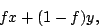in other words, to a weighted average of the previous and the new value, whose weights favor the new value more if the time of the sporadic value is earlier, closer to. In the example shown, the transition from 0 to 1 at time 2 giveswhile the transition from 1 to 0 at time 4.75 gives:This technique gives a still closer representation of the control signal (at least, the portion of it that lies below the Nyquist frequency), at the expense of more computation and slightly greater delay.

Numeric control streams may also be converted to audio signals using ramp functions to smooth discontinuities. This is often used when a control stream is used to control an amplitude, as described in Section 1.5. In general there are three values to specify to set a ramp function in motion: a start time and target value (specified by the control stream) and a target time, often expressed as a delay after the start time.

In such situations it is almost always accurate enough to adjust the start and ending times to match the first audio sample computed at a later logical time, a choice which corresponds to the fast-as-possible scenario above. Figure 3.5 (part a) shows the effect of ramping from 0, starting at time 3, to a value of 1 at time 9, immediately starting back toward 0 at time 15, with block size. The times 3, 9, and 15 are truncated to 0, 8, and 12, respectively.In real situations the block size might be on the order of a millisecond, and adjusting ramp endpoints to block boundaries works fine for controlling amplitudes; reaching a target a fraction of a millisecond early or late rarely makes an audible difference. However, other uses of ramps are more sensitive to time quantization of endpoints. For example, if we wish to do something repetitively every few milliseconds, the variation in segment lengths will make for an audible aperiodicity.

For situations such as these, we can improve the ramp generation algorithm to start and stop at arbitrary samples, as shown in Figure 3.5 (part b), for example. Here the endpoints of the line segments line up exactly with the requested samples 3, 9, and 15. We can go even further and adjust for fractional samples, making the line segments touch the values 0 and 1 at exactly specifiable points on a number line.

For example, suppose we want to repeat a recorded sound out of a wavetable 100 times per second, every 441 samples at the usual sample rate. Rounding errors due to blocking at 64-sample boundaries could detune the playback by as much as a whole tone in pitch; and even rounding to one-sample boundaries could introduce variations up to about 0.2%, or three cents. This situation would call for sub-sample accuracy in sporadic-to-audio conversion.Next: Converting from audio signals Up: Audio and control computations Previous: Control   Contents   Index
Miller Puckette 2006-12-30